# FORM¶

The First Order Reliability Method is used under the following context: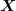is the input random vector and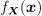its joint density probability function.
Let us denote by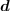a deterministic vector,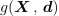the limit state function of the model,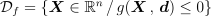the event considered here and g(,) = 0 its boundary.
The objective of FORM is to evaluate the probability content of the event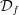:

(1)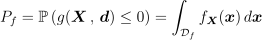The method proceeds in three steps:

1. Map the probabilistic model in terms ofthanks to an isoprobabilistic transformation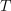which is a diffeomorphism from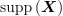into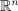, such that the distribution of the random vector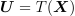has the following properties :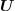and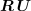have the same distribution for all rotations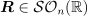.
The usual isoprobabilistic transformations are the Generalized Nataf transformation and the Rosenblatt one.
The mapping of the limit state function is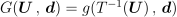. Then, the event probability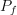rewrites :

(2)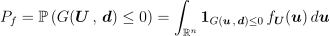where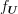is the density function of the distribution in the standard space : that distribution is spherical (invariant by rotation by definition). That property implies thatis a function of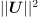only. Furthermore, we suppose that outside the sphere which tangents the limit state surface in the standard space,is decreasing.

2. Find the design point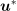which is the point verifying the event of maximum likelihood : the decreasing hypothesis of the standard distributionoutside the sphere which tangents the limit state surface in the standard space implies that the design point is the point on the limit state boundary the nearest to the origin of the standard space. Thus,is the result of a constrained optimization problem.
3. In the standard space, approximate the limit state surface in the standard space by a linear surface at the design point. Then, the probability((2)) where the limit state surface has been approximated by a linear surface (hyperplane) can be obtained exactly, thanks to the rotation invariance of the standard distribution:

(3)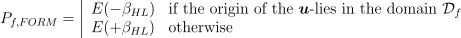where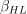is the Hasofer-Lind reliability index, defined as the distance of the design pointto the origin of the standard space and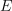the marginal cumulative density function of the spherical distributions in the standard space.
Let us recall here that in the Rosenblatt standard space, random vectors follow the standard normal distribution (with zero mean, unit variance and unit correlation matrix), which implies that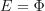. In the Generalized Nataf standard space, random vectors follow some spherical distributions, with zero mean, unit variance, unit correlation matrix and which type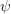is the one of the copula of the physical random vector: in that case,is the 1D cumulative distribution function with zero mean, unit variance and which type is.

Here, the event considered is explained directly from the limit state function: this is the classical structural reliability formulation. However, if the event is a threshold exceedance, it is useful to explicit the variable of interest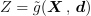, evaluated from the model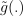. In that case, the event considered, associated to the threshold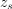has the formulation: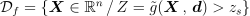and the limit state function is :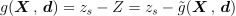.is the threshold exceedance probability, defined as :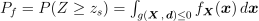.